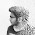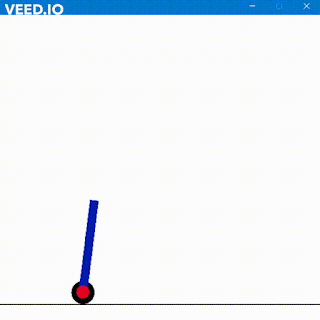When full adders are used they often introduce a time delay since every single bit addition depends on the carry of previous addition. Hence C4 will wait for C3 and C3 will for C2 and so on.

A carry look ahead adder basically reduces the time complexity however increases the gate complexity as well as space complexity, hence its cost always increases. In CLA adder a concept comes of Generate Carry and Propagate Carry. The Generate Carry is produced when both of the A and B are 1 and it doesn’t depend on Ci at that moment.

The Propagate Carry is produced when atleast one of A and B is 1 or whenever there is an input carry then the input carry is propagated.

But why do we have “LOOK AHEAD” here. It is because whenever two bits are gonna be added then Generate and Propagate will determine whether the carry will generate of input carry will propagate. Thus when no Propagation is required addition takes place without waiting for the ripple carry

Thus to determine Generate we have
Gi = Ai . Bi
To determine Propagate we have
Pi = Ai EXOR Bi

Thus the basic equation comes in detail as
Ci+1 = Gi + Pi . Ci
which gives all C,C,C,C as
C = Cin
C = G + ( P & C )
C = G + ( P & G ) + ( P & P & Cin )
C = G + ( P & G ) + ( P & P & G ) + ( P & P & P & Cin );
Cout = G+ ( P & G ) + ( P & P & G ) + ( P & P & P & G ) + ( P & P & P & P & C )

The sum is concat of Cout and P EXOR C
S = {Cout,P^C}

The Group Generate is
GG = P & P & P & P
The Propagate Group is
PG = G | (P & G) | (P & P & G) | (P & P & P & G);

When multiple CLA's are joined then the GG and PG of each group is used to determine input carry for the CLA's.

Code for 4 bit CLA

module CLA(a,b,ci,co,s);
input [3:0]a,b;
output [4:0]s;
input ci;
output co;
wire [3:0]G,P,C;
assign G = a&b;
assign P = a^b;
assign co=G+ (P&G) + (P&P&G) +  (P&P&P&G) + (P&P&P&P&ci);
assign C=G + (P&G) + (P&P&G) +  (P&P&P&ci);
assign C=G + (P&G) + (P&P&ci);
assign C=G + (P&ci);
assign C=ci;
assign s = {co,P^C};
endmodule

Code for Test Bench
module fi;
reg [3:0] a;
reg [3:0] b;
reg ci;
wire co;
wire [4:0] s;
CLA uut (
.a(a),
.b(b),
.ci(ci),
.co(co),
.s(s)
);

initial begin
// Initialize Inputs
a = 4'b1101;
b = 4'b1011;
ci = 0;
end

endmodule

Here is the output of CLA

v

1.test bench was not working

1.Working for me here
Do check that module name and instantiated module name inside test bench is same

My module name here is CLA

2.can you please provide CLA using PGcells

3.